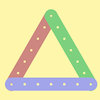#### You may also like### I'm Eight

Find a great variety of ways of asking questions which make 8.### Let's Investigate Triangles

Vincent and Tara are making triangles with the class construction set. They have a pile of strips of different lengths. How many different triangles can they make?### Noah

Noah saw 12 legs walk by into the Ark. How many creatures did he see?

# Even and Odd

##### Age 5 to 7Challenge Level

This fun problem was about odd and even numbers; it also involved some counting, which is always a good thing! From this specific problem, some general rules began to emerge. This is very useful as these rules can be applied to other problems.

Matthew and Hannah from Stambridge submitted a lovely and clear solution:

Even numbers have two rows of the same number of blocks.

Odd numbers have one extra block in one of the rows.

When you add an odd number of blocks to an even number you get an odd number.

When you add an even number of blocks to an even number you get an even number.

When you add an odd number of blocks to an odd number you get an even number.

Class 4SK from Devonshire Primary also submitted a good solution:

E= Even and O= Odd

E+E=E
O+O=E
O+E=O
three odd numbers added = an odd number

They also explained why this is the case:

An odd number is a number with one left when we pair them up. So if we add two odd numbers, the two left over can make a pair.

Well done too to children from St Andrews CE Primary School in Oxford whose solution was very similar to Class 4SK's.
Thank you and well done to all those who submitted solutions! If you enjoyed this, why not try the following problem?

If you are only allowed to use 1s, 3s and 5s (but as many of each as you like), can you find all the ways to make 12 using four numbers? Can you find all the ways to make 13 using four numbers?